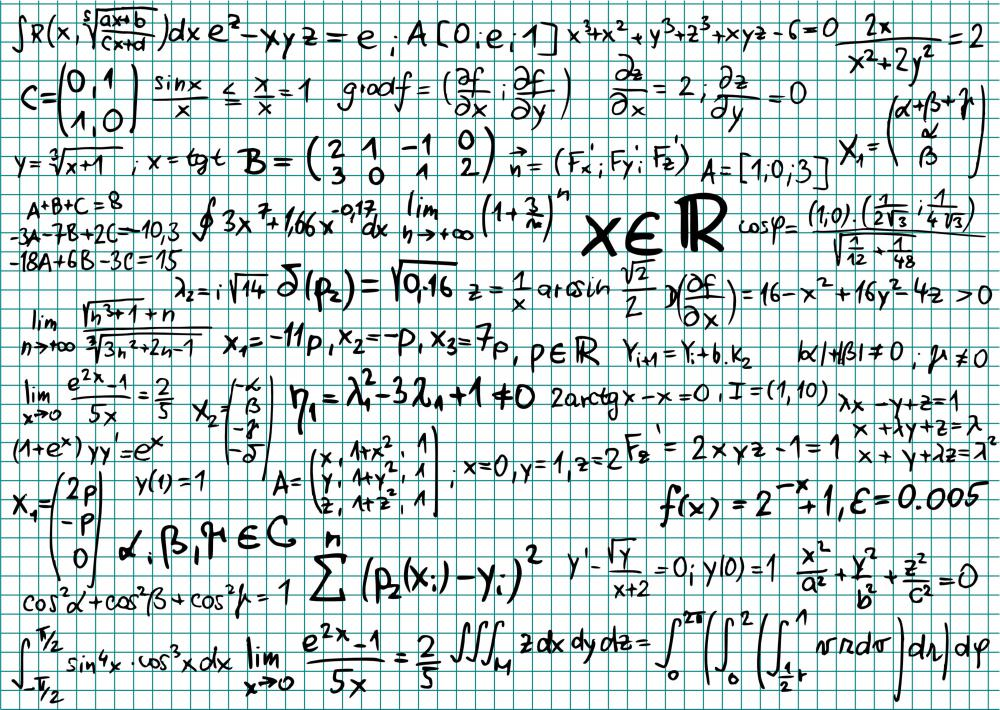# What Is Analysis of Variance?

A. Reed

When doing research, sometimes it becomes necessary to analyze data comparing more than two samples or groups. A type of inferential statistics test, analysis of variance (ANOVA), permits examination of several samples at the same time for purposes of determining whether a significant relationship exists between them. Reasoning is identical to t-tests, only analysis of variance includes independent variables of two or more samples. Differences between samples as well as the difference within one sample is determined. ANOVA is based upon four assumptions: the level of measurement, the sampling method, the distribution of the population, and the homogeneity of the variance.The standard deviation is more frequently used than variance because it is more intuitive and shares the same units as the mean.

In order to determine whether differences are significant, ANOVA is concerned with differences between and within the samples, which is referred to as the variance. The ANOVA can find out if the variance is larger between samples as compared to that among sample members. If this is found to be true, then the differences are considered to be significant.

Conducting an ANOVA test involves acceptance of certain assumptions. The first is that the independent random sampling method is used and the choice of sample members from a single population does not influence the choice of members from later populations. Dependent variables are measured primarily at the interval-ratio level; however, it is possible to apply the analysis of variance to ordinal-level measurements. One can assume that the population is normally distributed, even though this is not verifiable, and population variances are the same, which means that the populations are homogeneous.

The research hypothesis assumes that at least one mean is different from the others, but the different means are not identified as larger or smaller. Only the fact that a difference exists is predicted. The ANOVA tests for the null hypothesis, which means that there is no difference among all of the mean values, such that A = B = C. This requires setting the alpha, referring to the probability level where the null hypothesis will be rejected.

F-ratio is a test statistic used specifically for analysis of variance, as the F score shows where the area of rejection for the null hypothesis begins. Developed by statistician Ronald Fisher, the formula for F is as follows: F = between group variance estimate (MSB) divided by the within group variance estimate (MSW), such that F = MSB/MSW. Each of the variance estimates consists of two parts — the sum of squares (SSB and SSW) and degrees of freedom (df). Using the Statistical Tables for Biological, Agricultural and Medical Research, the alpha can be set and based upon this, and the null hypothesis of no difference can be rejected. It can be concluded that a significant difference exists between all of the groups, if that is the case.

## You might also Like